`What products are involved in this article?This article is based on the use of RCP (Rheonics Control Panel) connected to the SME (Smart Module Electronics) operating SRD, DVP, and DVM. Hence it can be used for any of these Rheonics sensors.`
`What is the purpose of this article?Explain the process to get a computed %w or %v (alcoholic strength by mass or volume) for ethanol-water from line density and temperature, using an iterative technique. Usual applications are with fluids with Ethanol-water mixtures.`

`1. Math model`

Shown below is the mathematical formula for OIML Concentration.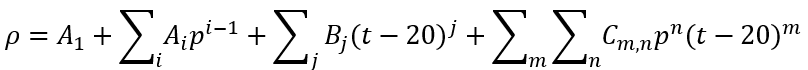Equation 1. OIML Concentration

The sensor can measure inline density, viscosity, and temperature, in addition, we can use multiple mathematical models to calculate the concentration of a mix. To calculate the OIML Concentration we can use Equation 1, where:

ρ: Density of ethanol/water mixture in Kg/m3.

p: Ethanol concentration by weight.

T: Temperature °C.

A, B, C: Coefficients.

i: 2 to 12.

j: 1 to 6.

m: 1 to 5.

n: 1 to 11.

`2. Checking the data`

All density, viscosity compensation, and concentration models can be loaded onto the sensor through the RCP software from Rheonics, in the “Calculation” tab. In this particular case, the model only applies to mixtures between alcohol and water, and all the values that you need to run the model are pre-loaded into the RCP Software. There are two ways to establish the concentration: by the Weight (%w) or through the Volume (%v).

In the chemical field, concentrations are often reported in %weight/weight (%w)or %mass/mass, (%m) some examples are Cetyl alcohol (C16H33OH), Isopropyl alcohol(C3H8O), and Benzyl alcohol (C3H8O).

Meanwhile, in the industry, the standard is %volume/volume (%v). The most common is the alcoholic beverage industry. Some example fluids are Wine, Beer, Rum, and Whiskey.

It should be noted that the type of concentration depends on each application and the standards that the user defines.

`3. How to load models into the Sensor electronics?`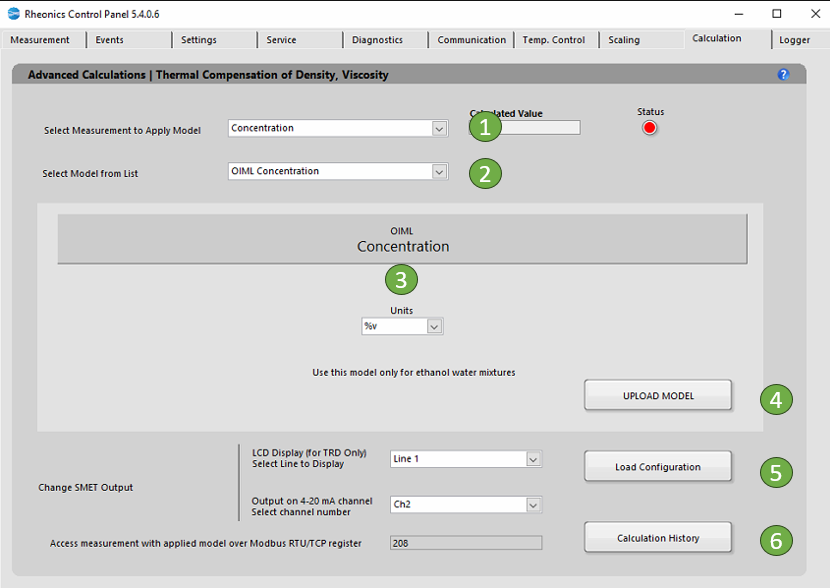Figure 1. Calculation tab for OIML Concentration

In the following steps, we will show how to load the model into the sensor electronics:

1. In the “Select Measurement to Apply Model” dropdown list select “Concentration”.

2. In the “Select Model from the list” dropdown list select “OIML Concentration“. When you select the model, you will get more information about the specific equation used and the coefficients you will need.”

3. The "Units" allow you to calculate concentrations based on mass (%w) or volume (%v).

4. Click “Upload Model” to load the model settings into sensor electronics. The button should turn GREEN for a couple of seconds if the action was successful or turn RED if the operation was unsuccessful. This action will refresh the “Calculated Value”.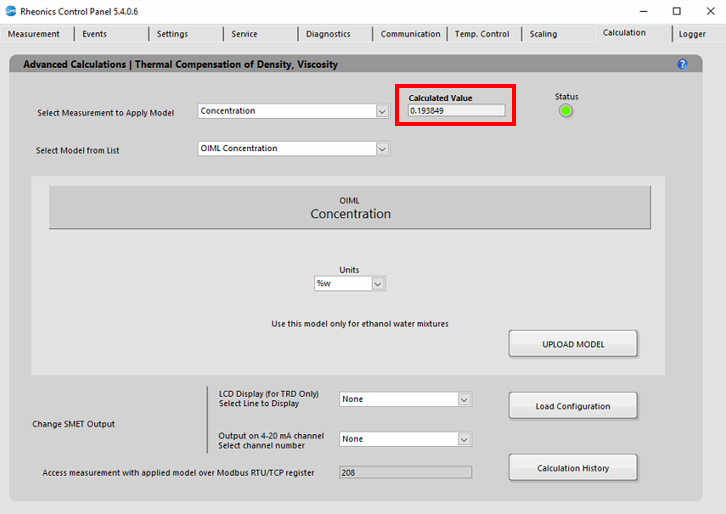Figure  2. Specific Gravity calculated

5. Click “Load Configuration” to update the display and channel configurations in sensor electronics.

6. The “Calculation History” button allows you to open a file with the last uploaded models.

3.1. Handling Sensor Outputs

For the SME-TRD, the compensated viscosity values can be shown on the display. Select the line to display the parameter: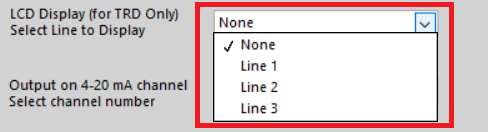Figure 4. Selecting read line for LCD display.

Select the analog channel to send data, this can be verified in the “Service” Tab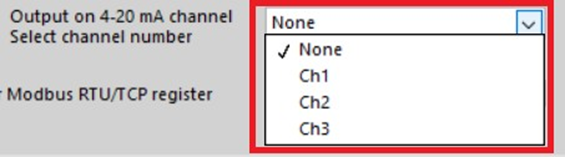Figure 5. Selecting analog output channel

Measurement can always be accessed at the register address that is displayed.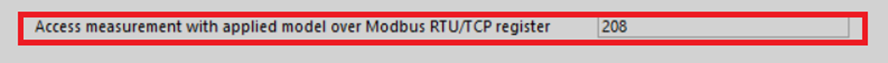Figure 6. Modbus TCP/IP register

3.2 Demonstration Video

Video 1. Configuration OIML Concentration model in RPC Software